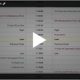# Treasuries Hedging and Risk Management

Interest Rate Education Homepage

Hedging interest rate risk with CME Group U.S. Treasury futures begins with identifying the futures contract’s CTD security. Once identified, we can determine the implied basis point value (BVP). BPV is also known as value of a basis point (VBP) or dollar-value of an .01 (DV01). They all refer the same thing, the financial change of the security or portfolio to a change in a 0.01% change in yield. To construct the proper dollar-weighted hedge ratio versus the product or position at risk we need to first determine the BPV.

Calculating Basis Point Value

The calculation for the BPV is simple: the contract’s CTD BPV divided by the CTD conversion factor (CF).

BPVcontract = BPVctd ÷ CFctd

Once we have the BPV, all we need is the BPV at risk.

Example

Assume you are long \$100 million of a U.S. Treasury portfolio with an average BPV of \$450 per million. This BPV is closest to the BPV of the CME Group U.S. Treasury 5-Year Note futures contract so we will use it as our hedging instrument.

The CTD for the 5-Year contract versus the March 2017 expiry is the 1.375% of May 31, 2021. It has a BPV of 42.45 per \$100,000 face value and a conversion factor of 0.8317.

We use \$100,000 because that is face value of one 5-Year Note futures contract. Our risk position is quoted in million-dollar increments so we will  make a slight multiplication to adjust apples for apples.

For our example, we have the following: BPVcontract = 42.45 / 0.8317 = \$51.04

The next step is to determine the value at risk. Our portfolio was \$100 million and the average BPV per million was \$450. Therefore, 450 x 100 = \$45,000 value at risk.

Now we can calculate our hedge ratio. We will use the following formula:

Hedge ratio (HR) = Value at risk ÷ Value of contract, or

HR = BPVrisk ÷ BPVcontract

HR = 45,000 / 51.04 = 881.66 or 882 5-Year futures

Because we are hedging a long position that is exposed to higher interest rates we would sell the futures contracts.

It would be highly unlikely for a portfolio manager to hedge her entire risk position. That would effectively leave her with no rate exposure. In other words, if rates went lower, she would not participate in the capital gain of higher prices. Usually risk managers of large rate positions use futures contracts to hedge a portion of their risk or to modify their portfolio’s target duration.

If you have questionsor schedule an online review .

Regards,

—————————————————————-

Privacy Notice

Disclosure